Example Questions

Example Question #451 : Arithmetic

John's shadow is six feet long, and Mary's shadow is five feet long. If John is four feet tall, which of the following is closest to Mary's height in inches?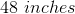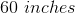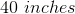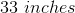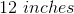Explanation:

First we set up a proportion. Mary's shadow (5 feet) to John's shadow (6 feet) is equal to Mary's height (x feet) to John's height (4 feet), i.e. 5 / 6 = x / 4.

Solve for x by first cross-mulitplying: 20 = 6x.

Divide both sides by 6: x = 20 / 6 feet

Multiply by 12 to find this height in inches: 20 * 12 / 6 = 20 * 2 = 40 inches

Example Question #1 : Compound Fractions

When two resistors (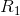and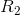) are added together in a parallel circuit to create an equivalent single resistor, the equivalent resistor (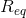) has a resistance defined by the following equation: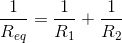If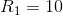and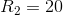, what doesequal?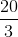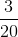Explanation:

Pluggingandinto the equation gives: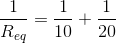Create a common denominator by multiplying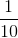byin the numerator and denominator: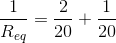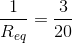Finally: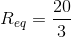Example Question #453 : Arithmetic

What does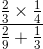equal?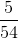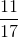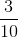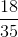Simplify the numerator first: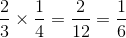.
Then, simplify the denominator: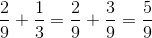.
Next, you have to do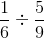, which is the same as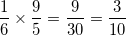, when solved for and simplified.